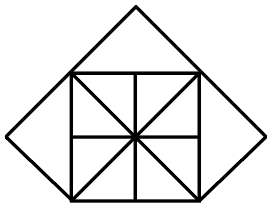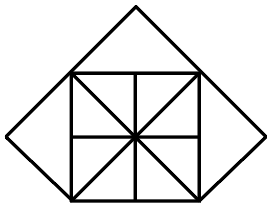Question 3

# How many triangles are there in the given figure?Solution

Given thatfrom the above figure, we count the triangle

then total triangle = 19 Ans

• Free SSC Study Material - 18000 Questions
• 230+ SSC previous papers with solutions PDF